# Point of Concurrency

Point of Concurrency

A carpenter designed a triangular table that had one leg. He used a special point of the table which was the center of gravity, due to which the table was balanced and stable.Do you know what this special point is known as and how do you find it?

This special point is the point of concurrency of medians.

This mini-lesson will also cover the point of concurrency of perpendicular bisectors, the point of concurrency of the angle bisectors of a triangle, and interesting practice questions.
Let’s begin!

## Lesson Plan

 1. What Is the Point of Concurrency? 2. Important Notes on the Point of Concurrency 3. Solved Examples on the Point of Concurrency 4. Challenging Questions on the Point of Concurrency 5. Interactive Questions on the Point of Concurrency

## What Is the Point of Concurrency?

The point of concurrency is a point where three or more lines or rays intersect with each other.

For example, referring to the image shown below, point A is the point of concurrency, and all the three rays l, m, n are concurrent rays.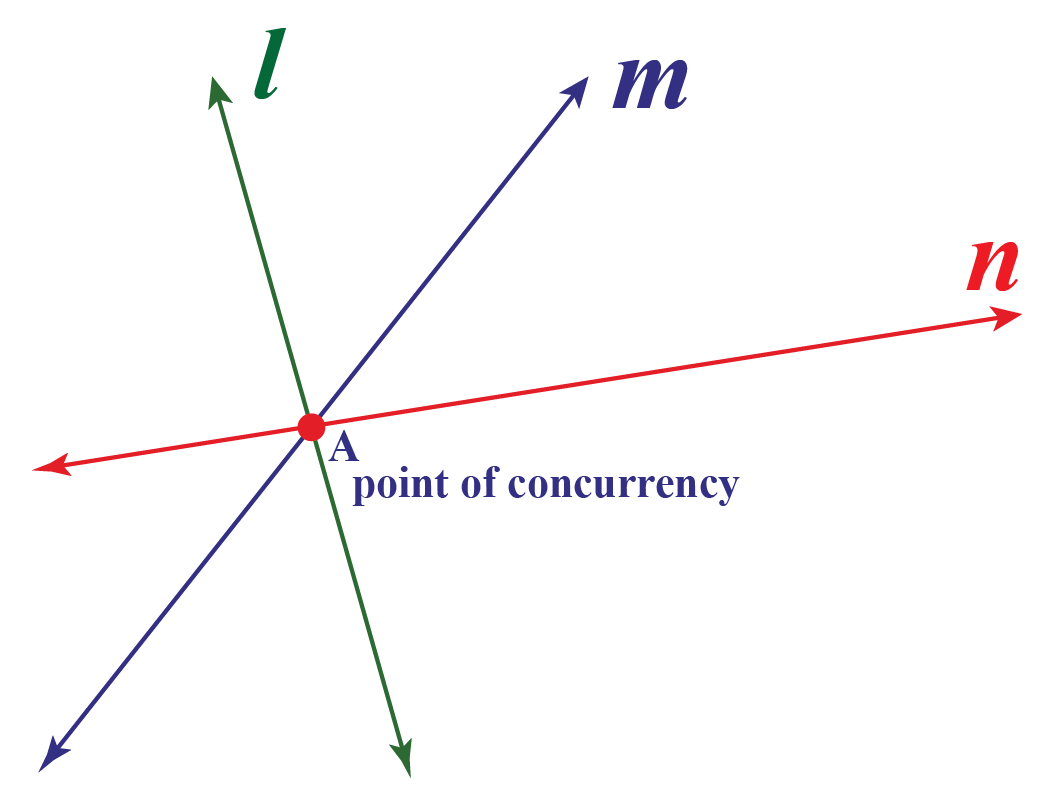## Triangle Concurrency Points

Four different types of line segments can be drawn for a triangle.

Please refer to the following table for the above statement:

Name of the line segment Description Example
Perpendicular Bisector These are the perpendicular lines drawn to the sides of the triangle.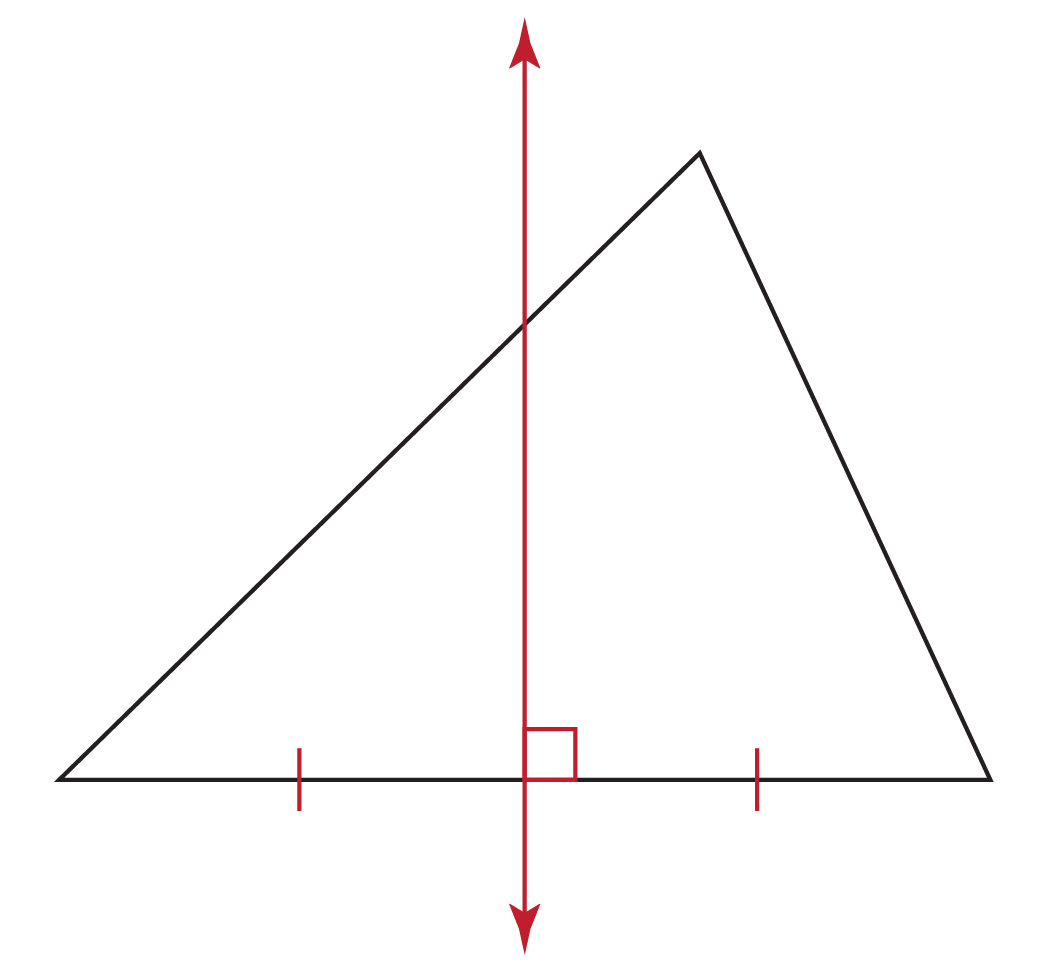Angle Bisector These lines bisect the angles of the triangle.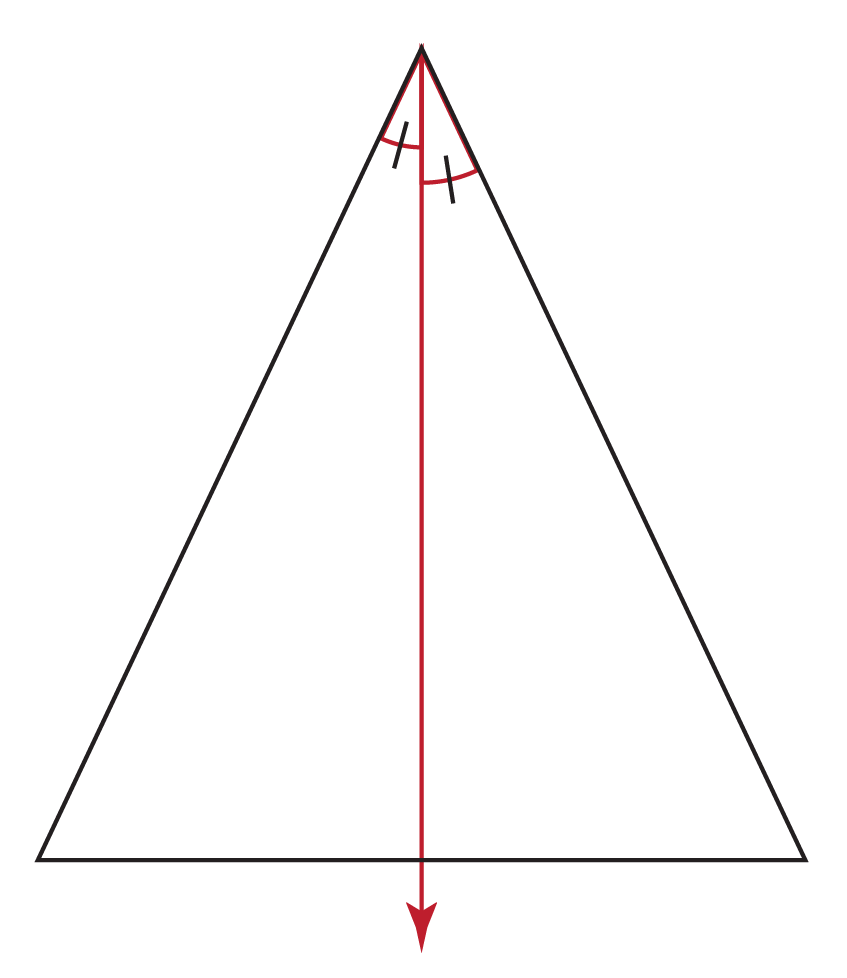Median These line segments connect any vertex of the triangle to the mid-point of the opposite side.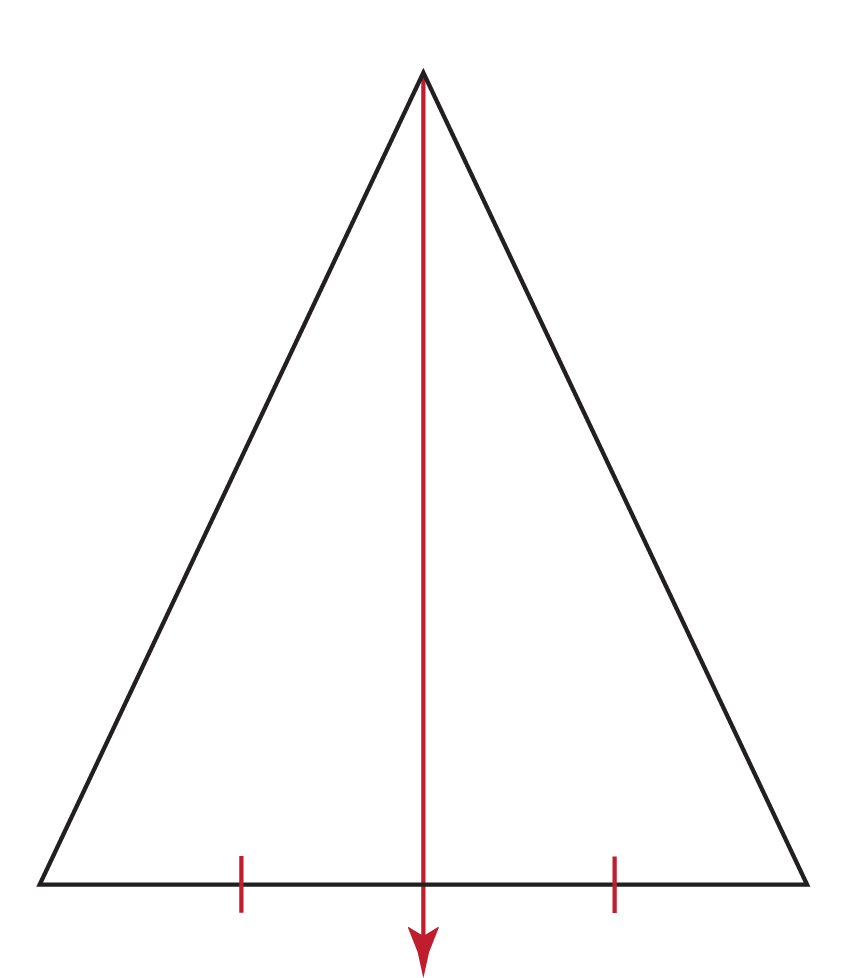Altitude These are the perpendicular lines drawn to the opposite side from the vertices of the triangle.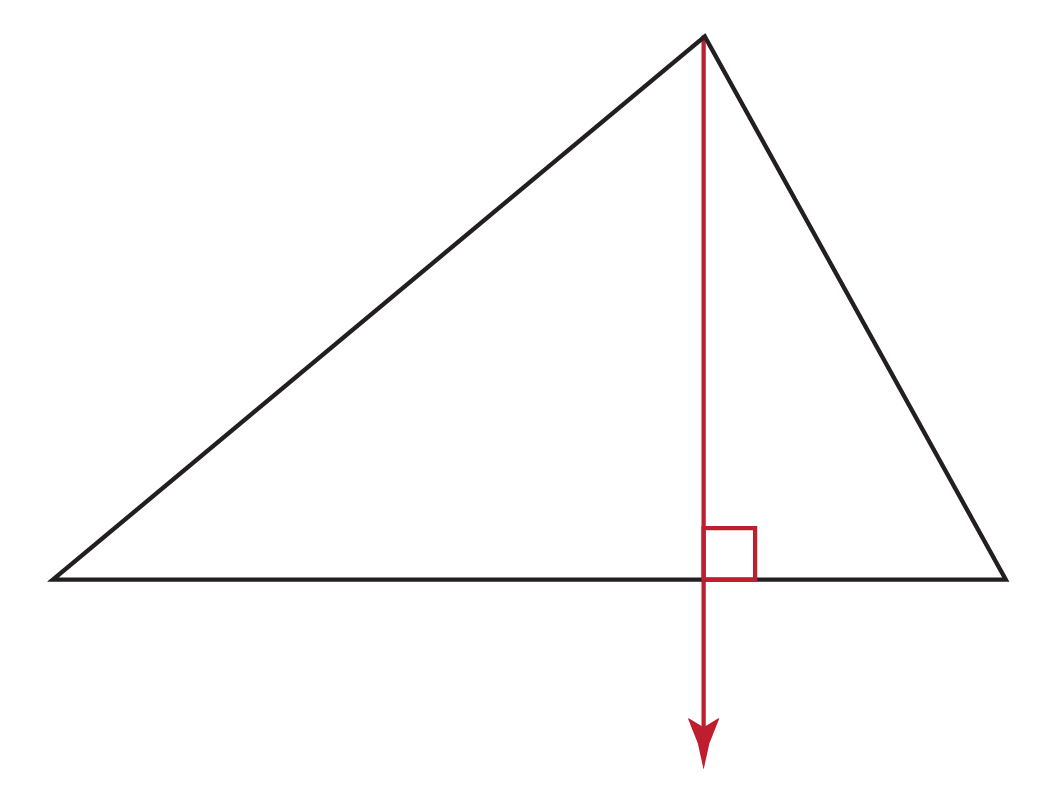As four different types of line segments can be drawn to a triangle, similarly we have four different points of concurrency in a triangle.

These concurrent points are referred to as different centers according to the lines meeting at that point.

The different points of concurrency in the triangle are:

1. Circumcenter.
2. Incenter.
3. Centroid.
4. Orthocenter.

1. Circumcenter

The circumcenter is the point of concurrency of the perpendicular bisectors of all the sides of a triangle.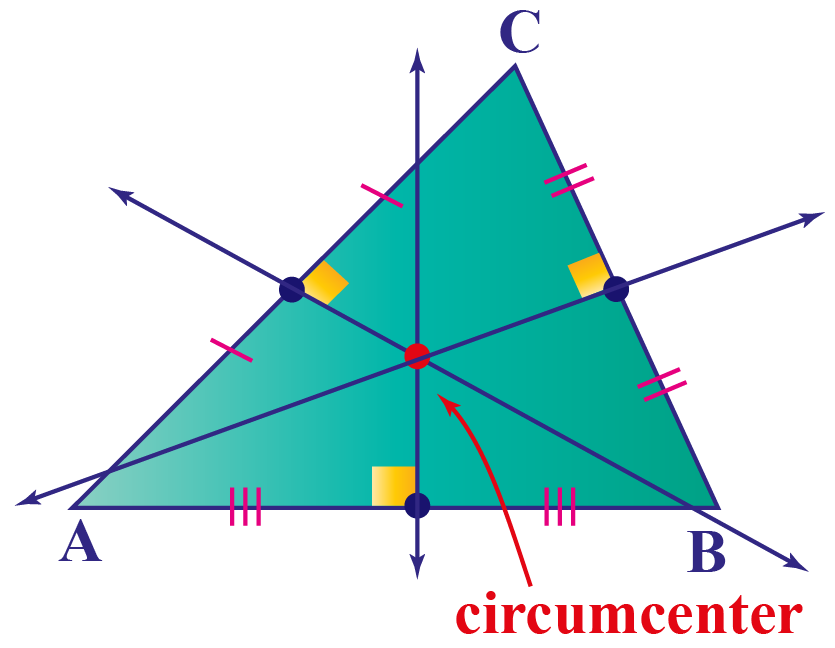For an obtuse-angled triangle, the circumcenter lies outside the triangle.

For a right-angled triangle, the circumcenter lies at the hypotenuse.

If we draw a circle taking a circumcenter as the center and touching the vertices of the triangle, we get a circle known as a circumcircle.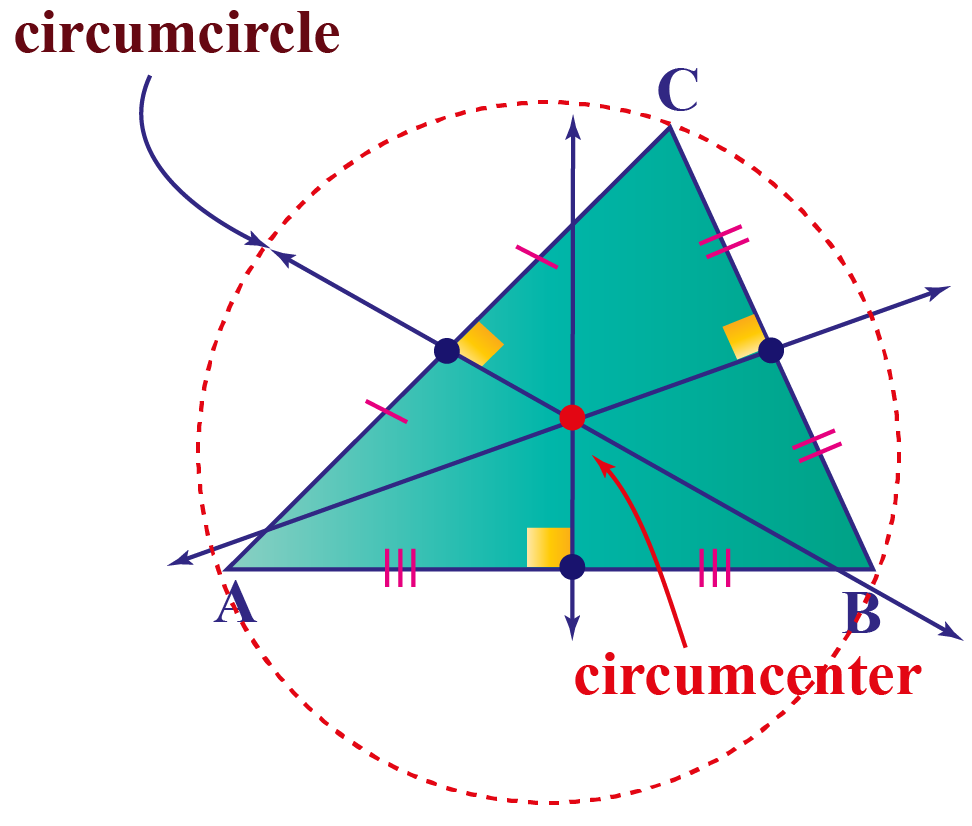2. Incenter

The incenter is the point of concurrency of the angle bisectors of all the interior angles of the triangle.

In other words, the point where three angle bisectors of the angles of the triangle meet are known as the incenter.

The incenter always lies within the triangle.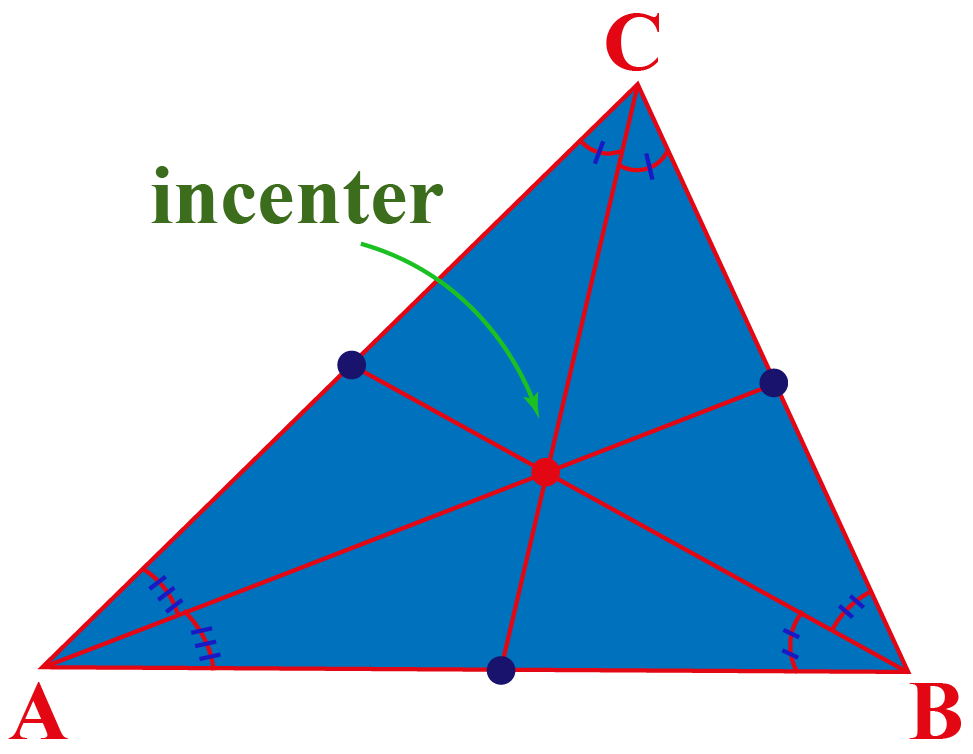The circle that is drawn taking the incenter as the center, is known as the incircle.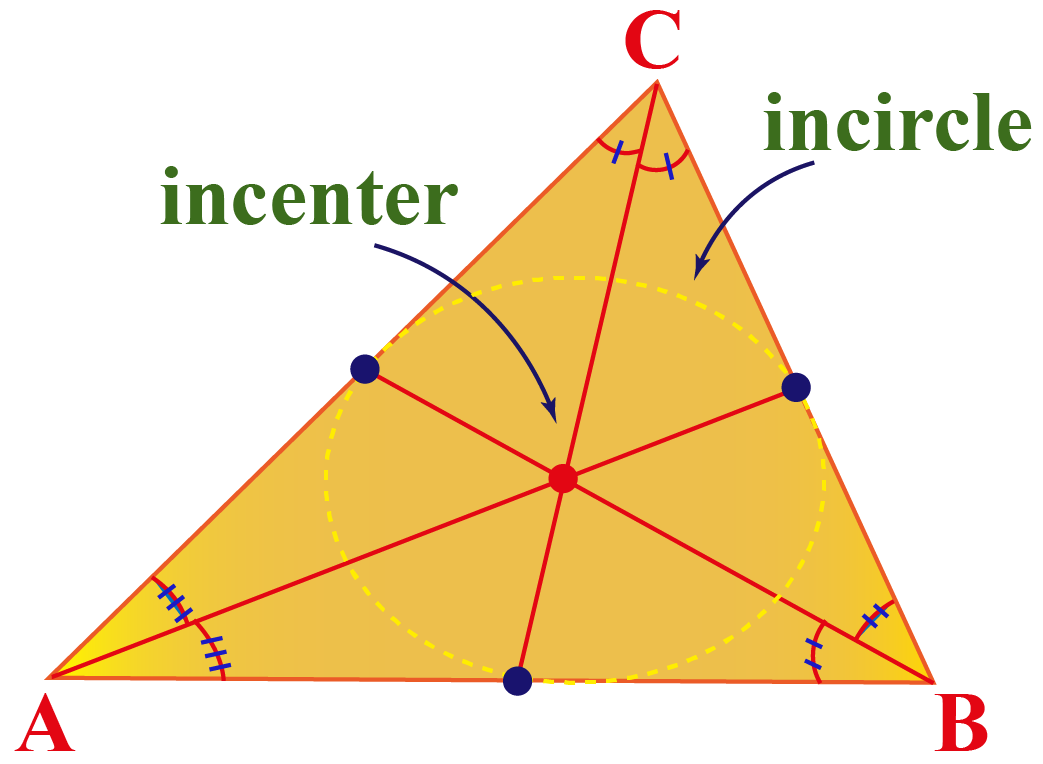3. Centroid

The point where three medians of the triangle meet is known as the centroid.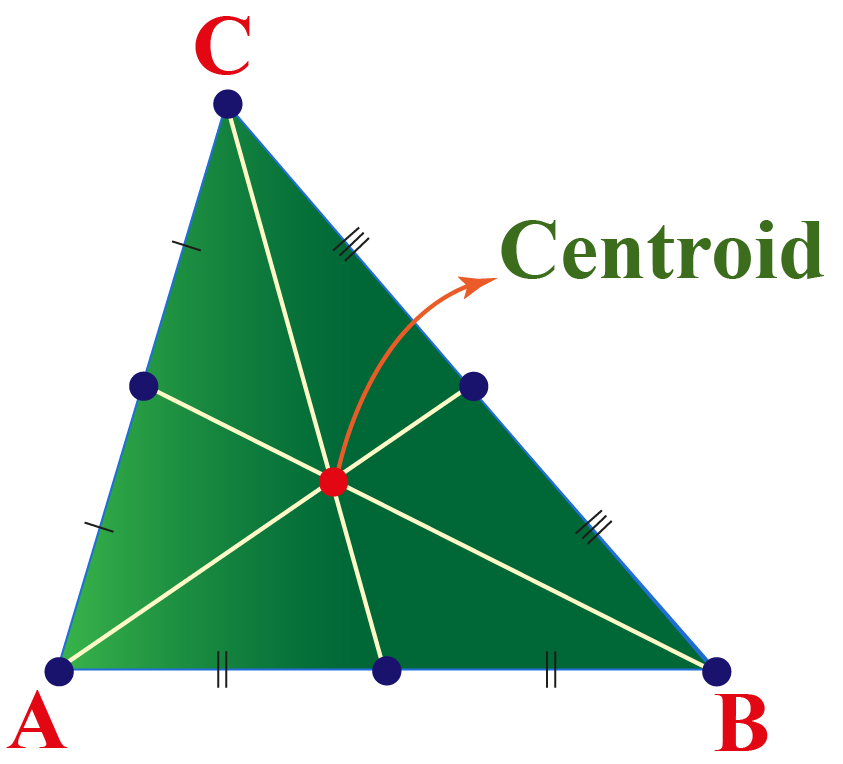In Physics, we use the term "center of mass" and it lies at the centroid of the triangle.

Centroid always lies within the triangle.

It always divides each median into segments in the ratio of 2:1.

4. Orthocenter

The point where three altitudes of the triangle meet is known as the orthocenter.

For an obtuse-angled triangle, the orthocenter lies outside the triangle.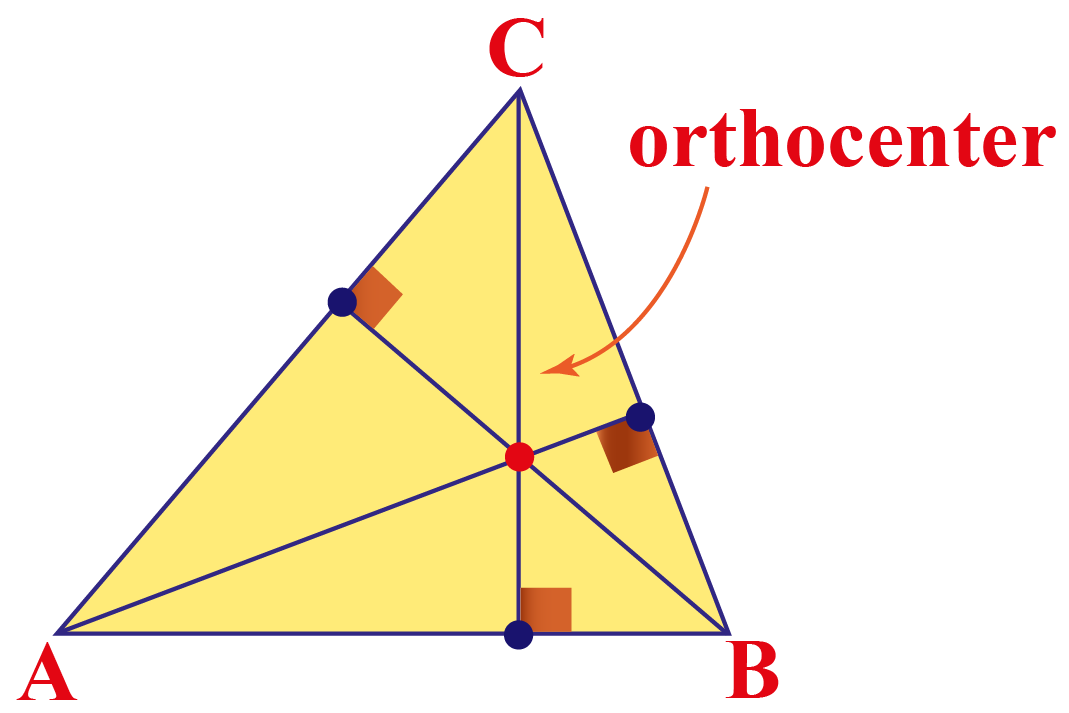Observe the different congruency points of a triangle with the following simulation:

More Important Topics
More Important Topics